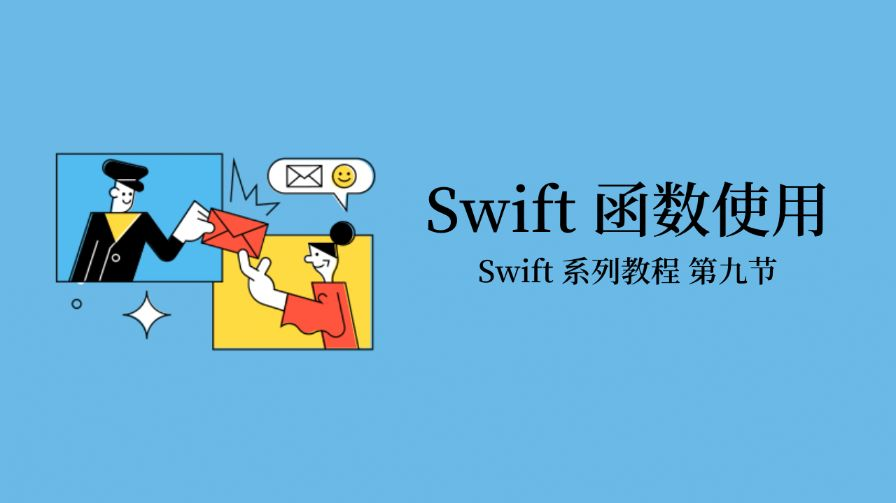#### Swift 函数使用``````func printName(m: String, n: String) {
print(m + n)
}

``````

func 是定义函数的关键字，printName 是函数名；

() 里面是可选参数列表，这里指定了 m 和 n 两个参数；

() 后面可以跟着函数的返回值，这里为空；

{ }里面封装了函数的逻辑，这里是调用了 print函数。

``````printName(m: "你好", n: "鸭")
``````

``````func minMax(array: [Int]) -> (min: Int, max: Int) {
var currentMin = array
var currentMax = array
for value in array[1..<array.count] {
if value < currentMin {
currentMin = value
} else if value > currentMax {
currentMax = value
}
}
return (currentMin, currentMax)
}

let bounds = minMax(array: [8, -6, 2, 109, 3, 71])

print("min is \(bounds.min) and max is \(bounds.max)")
``````

( ) 后面跟了函数的返回值，这里返回了两个 Int值。

{ }里面是函数执行的逻辑，这里循环遍历比较了传入的数组里的数字，得到了数组里最大和最小的两个值，当成函数的结果返回。